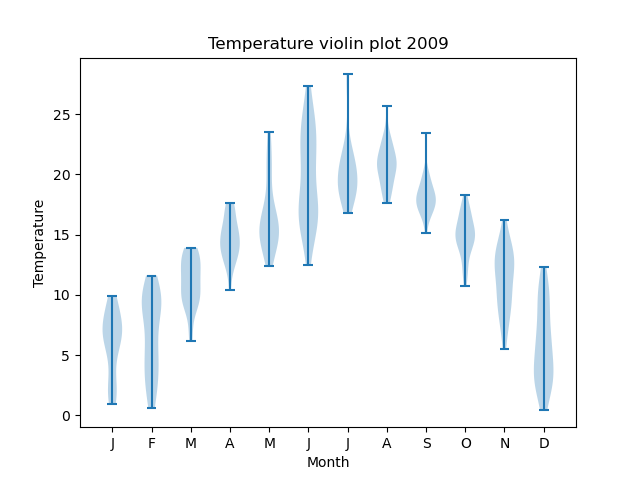# Violin plots in Matplotlib

Martin McBride, 2022-06-24
Tags matplotlib violin plot
Categories matplotlibA violin pot is similar to a box plot. The difference is that, for each data point, it displays a small histogram to show the data variance. This gives a bit more detail than a box plot, which only shows teh quartiles.

That doesn't automatically make violin pots better than box plots, because sometimes a box plot can be clearer and easier to read.

## Example violin plot

Here is an example:For a violin plot, the main line of each figure goes from the minimum value to the maximum value, including any outliers. The shaded area indicates the distribution of values within that range.

Here is the code:

import matplotlib.pyplot as plt
import csv

with open("2009-temp-monthly-list.csv") as csv_file:

month_names = ["J", "F", "M", "A", "M", "J",
"J", "A", "S", "O", "N", "D"]

months = range(12)

plt.title("Temperature violin plot 2009")
plt.xlabel("Month")
plt.ylabel("Temperature")
plt.violinplot(temperature, positions=months)
plt.xticks(months, month_names)
plt.show()


The code is here on github, in the file violinplot_temperatures.py.

The only change compared to the box plot code is the call to violinplot rather than boxplot.

If you found this article useful, you might be interested in the course Introduction to Matplotlib by the same author.

#### Popular tags

2d arrays abstract data type alignment and angle animation arc array arrays bar chart bar style behavioural pattern bezier curve built-in function callable object chain circle classes clipping close closure cmyk colour combinations comparison operator comprehension context context manager conversion count creational pattern data science data types decorator design pattern device space dictionary drawing duck typing efficiency ellipse else encryption enumerate fill filter font font style for loop function function composition function plot functools game development generativepy tutorial generator geometry gif global variable gradient greyscale higher order function hsl html image image processing imagesurface immutable object in operator index inner function input installing iter iterable iterator itertools join l system lambda function len lerp line line plot line style linear gradient linspace list list comprehension logical operator lru_cache magic method mandelbrot mandelbrot set map marker style matplotlib monad mutability named parameter numeric python numpy object open operator optimisation optional parameter or pandas partial application path pattern permutations pie chart polygon positional parameter print pure function python standard library radial gradient range recipes rectangle recursion reduce regular polygon repeat rgb rotation roundrect scaling scatter plot scipy sector segment sequence setup shape singleton slice slicing sound spirograph sprite square str stream string stroke structural pattern subpath symmetric encryption template text text metrics tinkerbell fractal transform translation transparency triangle truthy value tuple turtle unpacking user space vectorisation webserver website while loop zip